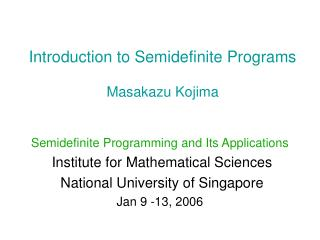DownloadDownload PresentationIntroduction to Semidefinite Programs Masakazu Kojima

# Introduction to Semidefinite Programs Masakazu Kojima

Télécharger la présentation## Introduction to Semidefinite Programs Masakazu Kojima

- - - - - - - - - - - - - - - - - - - - - - - - - - - E N D - - - - - - - - - - - - - - - - - - - - - - - - - - -
##### Presentation Transcript

1. Introduction to Semidefinite ProgramsMasakazu Kojima Semidefinite Programming and Its Applications Institute for Mathematical Sciences National University of Singapore Jan 9 -13, 2006

2. Main purpose • Introduction of semidefinite programs • Brief review of SDPs Contents Part I: Introduction to SDP and its basic theory --- 70 minutes Part II: Primal-dual interior-point methods --- 70 minutes Part III: Some applications Appendix: Linear optimization problems over symmetric cones References --- Not comprehensive but helpful for further study of the subject ---

3. Contents Part I: Introduction to SDP and its basic theory 1. LP versus SDP 2. Why is SDP interesting and important? 3. The equality standard form 4. Some basic properties on positive semidefinite matrices and their inner product 5. General SDPs 6. Some examples 7. Duality Part II: Primal-dual interior-point methods 1. Existing numerical methods for SDPs 2. Three approaches to primal-dual interior-point methods for SDPs 3. The central trajectory 4. Search directions 5. Various primal-dual interior-point methods 6. Exploiting sparsity 7. Software packages 8. SDPA sparse format 9. Numerical results

4. Part III: Some applications 1. Matrix approximation problems 2. A nonconvex quadratic optimization problem 3. The max-cut problem 4. Sum of squares of polynomials Appendix: Linear optimization problems over symmetric cones 1. Linear optimization problems over cones 2. Symmetric cones 3. Euclidean Jordan algebra 4. SOCP (Second Order Cone Program) 5. Some applications of SOCPs

5. Part I: Introduction to SDP and its basic theory1. LP versus SDP2. Why is SDP interesting and important? 3. The equality standard form4. Some basic properties on positive semidefinite matrices and their inner product5. General SDPs6. Some examples7. Duality

6. Part I: Introduction to SDP and its basic theory1. LP versus SDP2. Why is SDP interesting and important? 3. The equality standard form 4. Some basic properties on positive semidefinite matrices and their inner product5. General SDPs6. Some examples7. Duality

7. Part I: Introduction to SDP and its basic theory1. LP versus SDP2. Why is SDP interesting and important? 3. The equality standard form4. Some basic properties on positive semidefinite matrices and their inner product5. General SDPs6. Some examples7. Duality

8. Classification of Optimization Problems Convex Continuous Discrete Nonconvex 0-1 Integer LP & QOP Linear Optimization Problem over Symmetric Cone relaxation Polynomial Optimization Problem SemiDefinite Program | | | | Second Order Cone Program POP over Symmetric Cone Convex Quadratic Optimization Problem BilinearMatrix Inequality Linear Program

9. Part I: Introduction to SDP and its basic theory1. LP versus SDP2. Why is SDP interesting and important?3. The equality standard form4. Some basic properties on positive semidefinite matrices and their inner product5. General SDPs6. Some examples7. Duality

10. Part I: Introduction to SDP and its basic theory1. LP versus SDP2. Why is SDP interesting and important? 3. The equality standard form4. Some basic properties on positive semidefinite matrices and their inner product5. General SDPs6. Some examples7. Duality

11. Part I: Introduction to SDP and its basic theory1. LP versus SDP2. Why is SDP interesting and important? 3. The equality standard form 4. Some basic properties on positive semidefinite matrices and their inner product5. General SDPs6. Some examples7. Duality

12. Part I: Introduction to SDP and its basic theory1. LP versus SDP2. Why is SDP interesting and important? 3. The equality standard form4. Some basic properties on positive semidefinite matrices and their inner product5. General SDPs6. Some examples7. Duality

13. Part I: Introduction to SDP and its basic theory1. LP versus SDP2. Why is SDP interesting and important? 3. The equality standard form SDP 4. Some basic properties on positive semidefinite matrices and their inner product5. General SDPs6. Some examples7. Duality

14. Part II: Primal-dual interior-point methods1. Existing numerical methods for SDPs2. Three approaches to primal-dual interior-point methods for SDPs 3. The central trajectory4. Search directions5. Various primal-dual interior-point methods6. Exploiting sparsity7. Software packages8. SDPA sparse format9. Numerical results

15. Part II: Primal-dual interior-point methods1. Existing numerical methods for SDPs2. Three approaches to primal-dual interior-point methods for SDPs3. The central trajectory4. Search directions5. Various primal-dual interior-point methods6. Exploiting sparsity7. Software packages8. SDPA sparse format9. Numerical results

16. Part II: Primal-dual interior-point methods1. Existing numerical methods for SDPs2. Three approaches to primal-dual interior-point methods for SDPs3. The central trajectory4. Search directions5. Various primal-dual interior-point methods6. Exploiting sparsity7. Software packages8. SDPA sparse format9. Numerical results

17. Part II: Primal-dual interior-point methods1. Existing numerical methods for SDPs2. Three approaches to primal-dual interior-point methods for SDPs3. The central trajectory4. Search directions5. Various primal-dual interior-point methods6. Exploiting sparsity7. Software packages8. SDPA sparse format9. Numerical results